# What's the underlying frame of the Einstein's Field Equation?

• I
Mentor
I have the MTW in my library but it's more than 1000 pages thick, I think I'll go with the Wald.
If you have a more mathematically rigorous bent, Wald is probably a better choice anyway. There is lots of math in MTW, but in general their approach is less rigorous and more geared towards physical intuition.

BTW are you little biased towards mathematicians or is it just an impression of mine :)?
There's nothing wrong with math, but it's not physics. If you want to learn physics, you should learn it from physicists, just as if you want to learn math, you should learn it from mathematicians. Mathematicians are just as horrified at physicists' attempts to teach math as physicists are at mathematicians' attempts to teach physics.•dextercioby
Mentor
how the (at least initial) observations of the masses enters the picture, as in the solar system scenario I've brought up?
Please read my post #70 first before trying to respond to my description of the general case.

Mentor
how the (at least initial) observations of the masses enters the picture, as in the solar system scenario I've brought up?
Assuming you've read post #70, here goes.

To analyze the solar system, we make a number of restrictive assumptions to reduce the number of unknowns. In the simplest case, where we consider the Sun to be the only massive body and the planets to be test objects, and we assume the Sun to be non-rotating and therefore spherically symmetric, we have already reduced the number of unknown functions in the metric to two, and since we are assuming vacuum outside the Sun itself (and we're not trying to solve for the Sun's interior), we have no unknown functions at all in the SET, since all of its components are just zero. And we already know that in this case, there is just one unique solution of the EFE, the Schwarzschild solution, in which the metric components are just functions of ##r##, and there is really only one such function that has to be determined by solving the EFE, which turns out to be ##1 - 2M / r## (with ##M## the Sun's mass), since the same function appears in both ##g_{tt}## and ##g_{rr}## (just in the denominator of the latter). (The theorem that proves this is called Birkhoff's theorem, and the proof is discussed in the Insights article on it that I referenced.)

Notice that nowhere in any of this did any "initial conditions" appear. That's because this solution is static, so there is no need for any "initial conditions" for the solution; it's the same at all times. The initial conditions for the motion of the planets is only needed to solve the geodesic equation for the orbits of each planet, not to solve the EFE itself. (I already pointed this out to @cianfa in an earlier post.)

•Pyter
Mentor
Intuitively I thought that the very concept of "curvature" implied the embedding on a higher space.
It does not - you are confusing two very different concepts: intrinsic curvature and extrinsic curvature.

The surface of a two-dimensional sphere (such as the idealized surface of the earth) has intrinsic curvature. The interior angles of triangles sum to more than 180 degrees, the Pythagorean theorem does not in general work, there are no parallel "straight lines" (these are great circles) because any two will always intersect at two points, ... All of these curvature effects are observed without ever leaving the two-dimensional surface of the Earth and are calculated and observed without ever involving the third dimension. More formally, the Riemann tensor is non-zero everywhere (and note that this is a coordinate-independent statement). Indeed, the whole point of differential geometry is to provide a mathematical treatment of N-dimensional manifolds without embedding them in a higher-dimensional space.

Extrinsic curvature is what happens when we bend an N-dimensional surface through a higher-dimensional space without distorting its geometry. Everyone's favorite example is the cylinder formed by bending a two-dimensional sheet of paper through the third dimension and joining the edges to form a tube: the surface remains euclidean and the hypothetical two-dimensional inhabitants of the sheet of paper will find none of the locally measurable effects that we find on the surface of a sphere. Formally, the Riemann tensor is zero everywhere - no curvature.
If you only have a 1D space, you wouldn't have "curved" or "straight" lines, you'd only have "lines", because the curvature only appears in a 2D space or higher. Same thing for the 2D, and higher dimensions, surfaces.
It is true that a 1D space cannot have intrinsic curvature (it can have extrinsic curvature - just form a closed circle and as with the two-dimensional tube no local property is affected), that the curvature of a 2D surface is characterized by a single number at every point, and so forth. But this has nothing to do with embedding in a higher dimension; we get these results by looking at the degrees of freedom of the Riemann tensor for the N-dimensional space we're considering. Which brings us to...
After all, the curvature of a surface is the rate of change of its normal vector
This is just plain wrong and may underlie much of your confusion here. The intrinsic curvature of a surface is defined by the Riemann tensor, and the Riemann tensor is defined by the behavior of infinitesimal vectors parallel transported within the manifold; no additional dimension is involved. That "rate of change of the normal vector" applies to extrinsic curvature and is completely irrelevant to general relativity.

•Pyter
Pyter
No, it isn't. It's a global coordinate on the spacetime. The chart you are looking at is a global chart, not a local one.
We're talking about the same thing. What I call "local coordinates" are what you call "global coordinates, labels attached to the spacetime events". I'm referring to the coordinates, each one ##\in E_1##, that a "local" chart covering all the manifold (thus actually a global chart) maps to, according to this definition: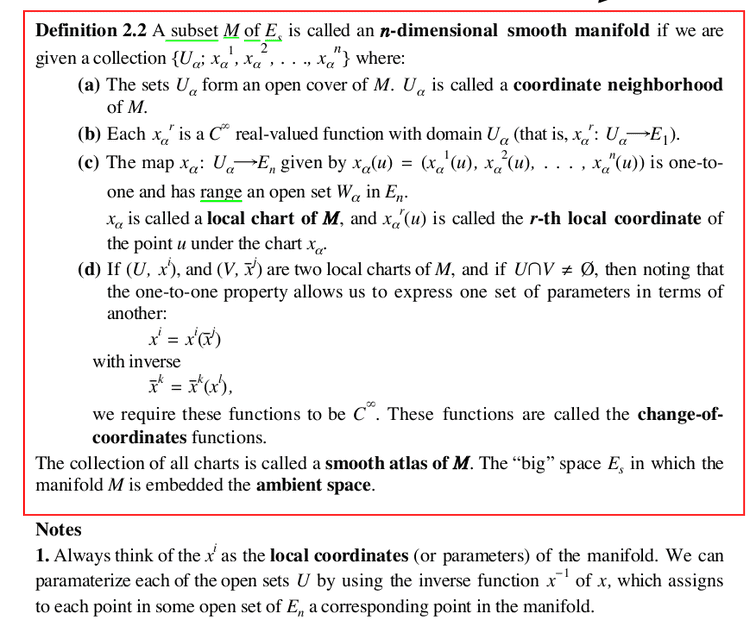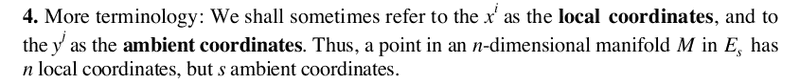To Waner's credit, he also notes that:@PeterDonis I think I've got the solar system case. The link between the physical observation and the SET is very simple.
What about a more complex case, where you have multiple comparable masses in the same region? What kind of constraints should the physical observations put on the EFE?

That "rate of change of the normal vector" applies to extrinsic curvature and is completely irrelevant to general relativity
Somebody should tell it to this author:
The Rhijk are the components of the Riemann curvature tensor. Note that the Riemann curvature tensor is defined in terms of the Christoffel symbols, which are functions of the metric coefficients. The Lij and Lij are functions of how the normal vector U changes, i.e., the curvature of the surface. Gauss defined the curvature of a 2-d surface geometrically, and showed that it equals det([L])/det([g]). The Riemann curvature tensor allows the curvature to be computed from values that can be calculated in the surface, the gij. It provides the building blocks for the left side of Einstein's field equations.

#### Attachments

•weirdoguy
Pyter
The intrinsic curvature of a surface is defined by the Riemann tensor, and the Riemann tensor is defined by the behavior of infinitesimal vectors parallel transported within the manifold; no additional dimension is involved.
Just for the records, I've checked Dirac's General Theory Of Relativity and he also seems to adopt the approach of the immersion in the N+1 dimensional space when explaining the parallel transport: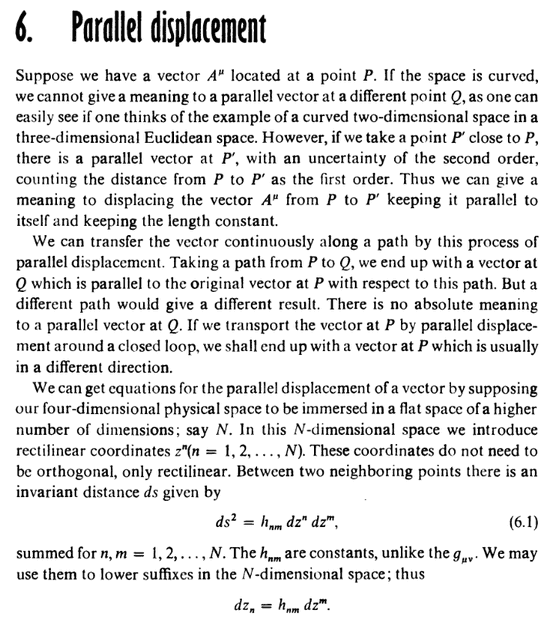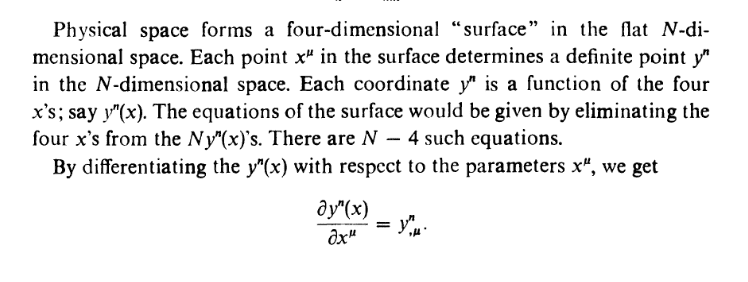•weirdoguy
Mentor
What I call "local coordinates" are what you call "global coordinates, labels attached to the spacetime events".
Ok. That means the reference you give is using terrible terminology, different from the literature on GR written and used by physicsts.

Somebody should tell it to this author:
No, somebody should, as I've already said, stop trying to learn physics from mathematicians. Physicists won't bother trying to tell the author this because none of them are going to read his book anyway. And mathematicians won't bother because they're not physicists and don't see the disconnect in the two bodies of literature.

That said, there is a key point that your reference fails to mention. It has chosen a particular embedding for the examples it gives of manifolds that makes the extrinsic curvature (the "rate of change of the normal vector") numerically equal to the intrinsic curvature (the Riemann tensor). But that does not make the two concepts identical, nor does it mean they will always be numerically equal for any embedding.

I strongly suggest that you take the time to read some references by physicists about GR, such as the ones we have already pointed out.

•vanhees71
Mentor
I've checked Dirac's General Theory Of Relativity and he also seems to adopt the approach of the immersion in the N+1 dimensional space when explaining the parallel transport:
Now check the references we gave you. What do they say?

Also note that Dirac does not say that ##N## dimensional curved spacetime is embedded in ##N + 1## dimensional flat space. He says that 4-dimensional spacetime can be treated as if it is embedded in ##N## dimensional flat space, without specifying what ##N## is. If you work it out, you will see that ##N## is not 4+1 in the case of 4-dimensional spacetime; it is greater (I think it works out to be 10 in the general case). Not all curved ##N## dimensional manifolds can be embedded in a flat ##N+1## dimensional space; that happens to be true for 2-dimensional manifolds but it does not generalize to higher dimensions.

•vanhees71 and dextercioby
Pyter
Now check the references we gave you. What do they say?
For instance Carroll says: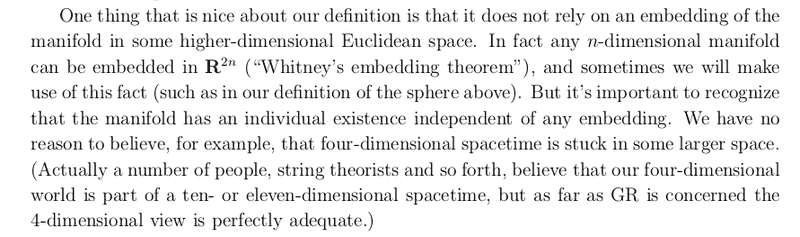I was citing Dirac because he's a physicist and he talks about manifolds embedded into higher dimension spaces, that you so much abhor. Just sayin'.
We all agree that the EFE is not expressed in ambient coordinates.

#### Attachments

•weirdoguy
Mentor
We all agree that the EFE is not expressed in ambient coordinates.
Yes, that was going to be the next thing I pointed out: that since your concern in this thread is solving the EFE, you should be looking at how all of the references discussed do that. They all do it in coordinates on the manifold itself, not making use of any embedding.

Mentor
I was citing Dirac because he's a physicist and he talks about manifolds embedded into higher dimension spaces, that you so much abhor.
My point all along is that if you want to solve the EFE, looking at embeddings is the wrong way to do it. Of course the embeddings your references talk about exist mathematically. That doesn't mean they're useful for doing physics.

•vanhees71
Homework Helper
As with Feynman and Weinberg's work on GR, Dirac's value of his 75 page brochure comes from treating GR as a classical field theory.

•Pyter and vanhees71
Gold Member
2022 Award
Besides, I really don't know how I could infer the general case from that special case.

Intuitively I thought that the very concept of "curvature" implied the embedding on a higher space.
If you only have a 1D space, you wouldn't have "curved" or "straight" lines, you'd only have "lines", because the curvature only appears in a 2D space or higher. Same thing for the 2D, and higher dimensions, surfaces.
After all, the curvature of a surface is the rate of change of its normal vector, and that is "outside" the surface, in a higher dimension space.
No! It's crucial to understand that you can define a Riemannian (or for GR a pseudo-Riemannian) manifold without any reference to an embedding in an higher-dimensional Euclidean (or pseudo-Euclidean) affine (flat) space. This has been found already by Gauss when he investigated 2D surfaces embedded in 3D Euclidean affine space: You don't need the embedding space to describe all the intrinsic properties of the surface, including distances and angles as well as curvature.

For a straight-forward introduction to GR, I'd recommend Landau&Lifshitz vol. 2. It contains the utmost minimum of tensor calculus you need to understand GR (Ricci calculus and tensor components wrt. holonomous coordinates) and concentrates on the physics. For the more refined mathematical aspects as well as more modern mathematical tools, you may look at Misner, Thorne, Wheeler, Gravitation.

•Klystron, Pyter, cianfa72 and 1 other person
Pyter
I've realized there's a big piece info I'm missing to get the whole picture: what coordinates system exactly is used for astronomical distances?

From my sparse notions, it should be a (quasi?)-Cartesian system with the z axis passing through the North Pole and the xy plane intersecting the Ecliptic line.
Of course a local chart in the tangent space can't be used because its Euclidean/Minkowskian approximation is only acceptable in a small region of the spacetime.

Does this frame take the curvature into account somehow?

Mentor
what coordinates system exactly is used for astronomical distances?
The usual ones are FRW coordinates, of which there are several different versions, described here:

https://en.wikipedia.org/wiki/Friedmann–Lemaître–Robertson–Walker_metric#General_metric

The usual ones are not Cartesian because our astronomical observations are of angular positions of objects on the sky, with distances estimated from those and other observations. So spherical coordinates of some kind (with several different possibilities for the radial coordinate) are much more suitable.

•vanhees71 and Pyter
Mentor
Does this frame take the curvature into account somehow?
Of course. Any valid coordinate chart on a curved spacetime that covers more than a local patch will have to take curvature into account in its expression for the metric.

•vanhees71
2022 Award
The usual ones are FRW coordinates,
That depends on what you mean by "astronomical distances". FRW is for very large distances (as I know you are well aware). We mostly just use good old Euclid for the solar system, probably with polar coordinates centered on something useful, or Sun-centered Schwarzschild if we need GR corrections. Presumably you'd use some modified Schwarzschild coordinates (or you could keep the coordinates and modify the metric) to model the solar system with planets as gravitational sources.

•vanhees71 and Pyter
Mentor
That depends on what you mean by "astronomical distances".
I had assumed that @Pyter meant distances on cosmological scales, but perhaps he can clarify. Or perhaps he meant both.•vanhees71
Mentor
We mostly just use good old Euclid for the solar system, probably with polar coordinates centered on something useful, or Sun-centered Schwarzschild if we need GR corrections.
AFAIK the usual solar system coordinates are barycentric, so all objects, including the Sun, move in these coordinates. The GR corrections are small enough in the solar system that you don't need the full Schwarzschild metric to model them; just the first few post-Newtonian orders is enough.

Presumably you'd use some modified Schwarzschild coordinates (or you could keep the coordinates and modify the metric) to model the solar system with planets as gravitational sources.
I believe that gravitational corrections due to the planets are modeled as Newtonian forces from point sources, not by altering either the solar system coordinates or the overall metric. The general framework used for these kinds of multi-body problems for weak fields is the Einstein-Infeld-Hoffman equations:

https://en.wikipedia.org/wiki/Einstein–Infeld–Hoffmann_equations

•vanhees71 and Pyter
Pyter
Of course. Any valid coordinate chart on a curved spacetime that covers more than a local patch will have to take curvature into account in its expression for the metric.
I gather that all these coordinates systems assume that the metric is already known?
For instance in the FRW the curvature is uniform everywhere:
whereranges over a 3-dimensional space of uniform curvature, that is, elliptical space, Euclidean space, or hyperbolic space.
so for "local" variations of curvature, like in a relative small region with several comparable masses, would it still be accurate?
our astronomical observations are of angular positions of objects on the sky, with distances estimated from those and other observations. So spherical coordinates of some kind (with several different possibilities for the radial coordinate)
Always in the case of many masses in a small region, wouldn't the radial coordinates be a little off because of the gravitational lens effect?
I had assumed that @Pyter meant distances on cosmological scales, but perhaps he can clarify.
I was thinking more of a relatively small region with comparable and meaningful masses, like maybe a neutron stars field, or just two orbiting one around the other.

Mentor
I gather that all these coordinates systems assume that the metric is already known?
No. If you would take the time to read the references you have been given, you would see how the coordinates are constructed using assumptions about the symmetries of the spacetime, before solving the EFE to find the full metric. You should realize by now that your current assumptions about how things are done are highly likely to be wrong, so you should stop making them.

For instance in the FRW the curvature is uniform everywhere
The spatial curvature is found to be uniform everywhere, but that is not an assumption, that is a deduction from other assumptions, made in the process of solving the EFE.

for "local" variations of curvature, like in a relative small region with several comparable masses, would it still be accurate?
The FRW metric is treated by cosmologists as an average description of the universe on large distance scales; it is not meant to capture variations on the scale of, say, the solar system, or even a small group of galaxies with empty space between them. The distance scale for the averaging is typically taken to be tens to hundreds of millions of light years.

There are papers in the literature investigating the possibility that variations in density (and hence curvature) on smaller scales might affect the large scale dynamics; so far that research is still open, but I think it's fair to say it has not yet produced any results that have convinced cosmologists in general.

wouldn't the radial coordinates be a little off because of the gravitational lens effect?
"A little off" in what sense? What would they be "off" from? Remember that the radial coordinate is not necessarily the same as radial distance.

I was thinking more of a relatively small region with comparable and meaningful masses, like maybe a neutron stars field, or just two orbiting one around the other.
For this case, the FRW solution is not what is used. There are no known exact solutions for isolated systems containing more than one gravitating mass (such as the binary pulsar systems that have given us evidence for gravitational wave emission); those kinds of cases can only be solved numerically.

•vanhees71
Pyter
No. If you would take the time to read the references you have been given, you would see how the coordinates are constructed using assumptions about the symmetries of the spacetime, before solving the EFE to find the full metric.
I meant: if the FRW is a "metric", that implies it's already a solution of the EFE which assumes a homogeneous distribution of the masses in the area where it's used.
The Friedmann–Lemaître–Robertson–Walker (FLRW; /ˈfriːdmən ləˈmɛtrə ... /) metric is an exact solution of Einstein's field equations of general relativity; it describes a homogeneous, isotropic, expanding (or otherwise, contracting) universe that is path-connected, but not necessarily simply connected.

Gold Member
2022 Award
Yes, and as was stressed before in this thread, that's a description of the "large-scale structure" of our universe, i.e., after "coarse graining" (i.e., averaging) over a large distance scale of a few 100 light years. It's based on the assumption of the "cosmological principle", which says that when averaging out the local "fluctuations" of the distribution of energy-momentum-stress of matter and radiation, the universe is homogeneous and isotropic, and this leads to the ansatz of maximally symmetric spacetimes and finally the FLRW solution of the EFE. This implies that on this level of description the energy-momentum-stress tensor is that of an ideal fluid.

Pyter
"A little off" in what sense? What would they be "off" from?
Excuse me, I meant the "angular" coordinate, off with respect to a situation without dense masses.

Mentor
if the FRW is a "metric" that implies it's already a solution of the EFE
The Wikipedia article uses the term "metric" to mean "general expression for a line element with unknown functions in it still to be determined by solving the EFE". That is clear from the context.

Also, in referencing the article, I clearly said "coordinates", and several different possible coordinate charts used in cosmology are described in the article, including the basics of how they are constructed without knowing the full metric (i.e., without having solved the EFE to find the unknown functions in the line element). The other references you have already been given (Carroll, MTW, Wald) all discuss in greater detail how all this is done.

Please read carefully before posting a knee-jerk reaction that does not correctly reflect what you were told.

Mentor
I meant the "angular" coordinate, off with respect to a situation without dense masses.
The observed angular coordinates can be "off" in this sense with gravitational lensing, yes. That's one of the things cosmologists have to try to correct for when constructing models.

Pyter
Also, in referencing the article, I clearly said "coordinates", and several different possible coordinate charts used in cosmology are described in the article, including the basics of how they are constructed without knowing the full metric
Even if the full metric is unknown, some assumptions on the mass distribution and thus on the EFE are made beforehand, and they're clearly not fulfilled in the neutron stars field scenario.
or this case, the FRW solution is not what is used. There are no known exact solutions for isolated systems containing more than one gravitating mass (such as the binary pulsar systems that have given us evidence for gravitational wave emission)
I thought so, the FRW, including the Cartesian coordinates, can't be used in this "extreme" case.

Mentor
Even if the full metric is unknown, some assumptions on the mass distribution and thus on the EFE are made beforehand, and they're clearly not fulfilled in the neutron stars field scenario.
Of course not. I explicitly said that the FRW assumptions apply to the universe as a whole.

the FRW, including the Cartesian coordinates, can't be used in this "extreme" case.
This isn't an "extreme" case, it's just a different case from modeling the universe as a whole. It's different because the assumptions are obviously different.

(Btw, there is nothing stopping you from using Cartesian coordinates in this case. The final metric obtained by numerically solving the EFE just won't look like an FRW metric in those coordinates.)

Pyter
This isn't an "extreme" case, it's just a different case from modeling the universe as a whole.
"Extreme" compared to the Schwa. metric, which can be solved in closed form without recurring to numerical methods.
Speaking of which, I think that what I wrote in post #46 of this thread wasn't so far fetched, at least in the Schwa. case.
Now I'm aware that both sides of the EFE contribute to ten differential equations whose unkown are the components of , and thus also the RHS depends on the linear coordinates through the unknown functions .
And as we saw the RHS does depends on g**, through T**.
The physical observation of your masses only set the boundary conditions of the EFE, and they contain the unknown in a parametrized form, for instance in the measurement of your distance from objects of known mass.
Maybe they don't set the boundary conditions, but at least an additional set of constraints helping solve the differential equations.
In Waner's op.cit., pag. 121, we can see that the observable quantity "total mass" helps solve for ##\Phi##: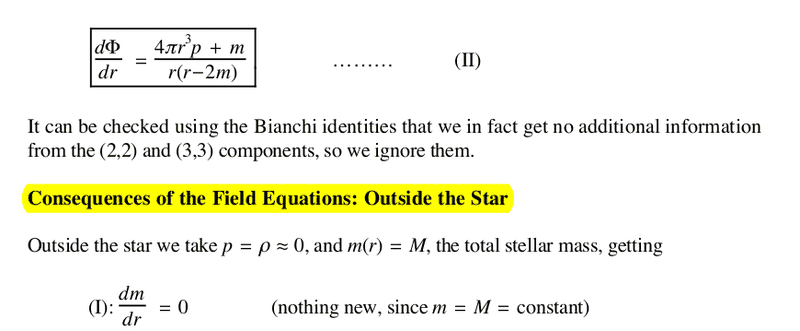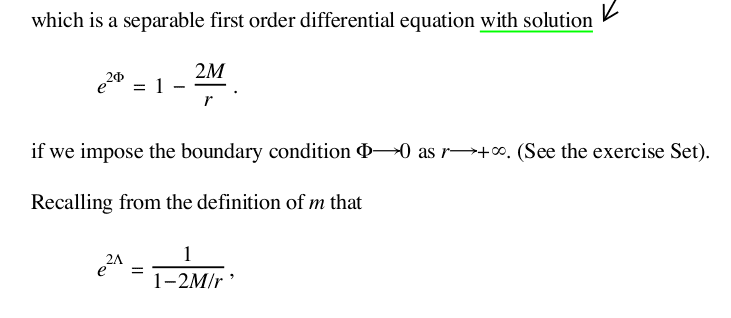I wonder it the above also applies to the neutron stars field or, to further simplify, the binary neutron star scenario? I'm not talking about actually solving the EFE, just setting it up.

Mentor
I think that what I wrote in post #46 of this thread wasn't so far fetched, at least in the Schwa. case.
Unfortunately, still no. See below.

Maybe they don't set the boundary conditions, but at least an additional set of constraints helping solve the differential equations.
The additional constraints in the Schwarzschild case are the symmetries of the spacetime, which drastically reduce the number of independent equations, plus the assumption of vacuum outside the star, which makes the differential equations homogeneous (zero on the RHS) and thereby further simplifies the solution. They have nothing to do with applying any boundary conditions or other conditions based on observation of the total mass.

In Waner's op.cit., pag. 121, we can see that the observable quantity "total mass" helps solve for ##\Phi##
No, it doesn't. The form of the solution comes from the drastic reduction in the number of independent equations due to symmetry. In the Schwarzschild case, this is what results in one of the EFE components becoming the separable first order equation with solution as described. The total mass ##M## is an undetermined constant in that solution, and it's known to be constant outside the star because outside the star is vacuum, not because knowing its actual value helps us in any way in solving the equations. (There is a boundary condition for ##\Phi##, that it should vanish as ##r \to \infty##, but this is true regardless of the actual value of the total mass ##M##, so knowing the total mass is irrelevant to applying it.)

I wonder it the above also applies to the neutron stars field
The general form given applies to any static, spherically symmetric mass distribution. One of my Insights articles that I referenced earlier in this thread discusses this (and also discusses how the symmetries simplify the solution).

or, to further simplify, the binary neutron star scenario?
The binary neutron star scenario is not simpler than a single neutron star; it's more complicated, and, as I have already said, no exact solution is known for it. Certainly you can't use a single Schwarzschild solution for it, since the scenario is neither static nor spherically symmetric. Not only that, but in the binary neutron star case, the field is too strong for nonlinearities to be ignored, so even the technique of simply adding together multiple Schwarzschild solutions centered on multiple massive objects does not work, since that technique assumes linearity.

•PeroK
Pyter
I wonder it the above also applies to the neutron stars field
@PeterDonis by the "above" I meant: 1) that the RHS depends on g**; 2) that the physical observations help reducing the degrees of freedom.

The first point for the Schwa. case is verified, the second maybe not because, as you said, due to the many symmetries, the equations are so simplified that the physical observations aren't even needed.

For the neutron stars field scenario — by that I mean a region with many neutron stars, and I called it sometimes the "general case" exactly because you can't rely on particular symmetries or uniform mass distribution hypothesis like in the "special" Schwa. case — I wondered if point 1) still holds. And if you can't simplify through symmetries, you're somewhat forced to use the physical observations to reduce the degrees of freedom, so I don't see why point 2) should not apply.

Mentor
@PeterDonis by the "above" I meant: 1) that the RHS depends on g**;
That's always true to the extent that the SET involves the metric.

2) that the physical observations help reducing the degrees of freedom.
As I have already said, what helps in reducing the degrees of freedom is assumptions about symmetries of the spacetime.

One could add to that assumptions restricting the form of the SET, which can reduce the number of unknown functions that must be solved for. The simplest of these is, of course, assuming vacuum; that means all SET components are zero. But we could also, for example, assume a perfect fluid with some particular equation of state (which is another common assumption for modeling, say, the interiors of stars and planets). In a sense assumptions like the latter are based on "physical observations", in that scientists have spend considerable time and effort figuring out useful equations of state for various phases of matter, but they're not observations of the particular system you're trying to model; they're just general observations that are useful background knowledge.

The first point for the Schwa. case is verified
It's always true for any case whatever.

the second maybe not because, as you said, due to the many symmetries, the equations are so simplified that the physical observations aren't even needed.
Correct. See above.

For the neutron stars field scenario — by that I mean a region with many neutron stars, and I called it sometimes the "general case" exactly because you can't rely on particular symmetries or uniform mass distribution hypothesis like in the "special" Schwa. case — I wondered if point 1) still holds.

And if you can't simplify through symmetries, you're somewhat forced to use the physical observations to reduce the degrees of freedom
But they don't. See above.

Pyter
But they don't. See above.
You mean that in the "general" (neutron stars) scenario, the physical observations (radial and angular positions, estimated (?) masses) are useless in setting up the EFE, let alone solve it?
Does it mean that in that case the EFE is not even solvable in theory?

Gold Member
2022 Award
Of course it is solvable, at least numerically. The really interesting question is about the correct equation of state of the strongly interacting matter the neutron star is made of. There is the hope to learn much more about it in the near future using gravitational wave observations as well as new experiments with heavy ions.

•Dale
Staff Emeritus
•